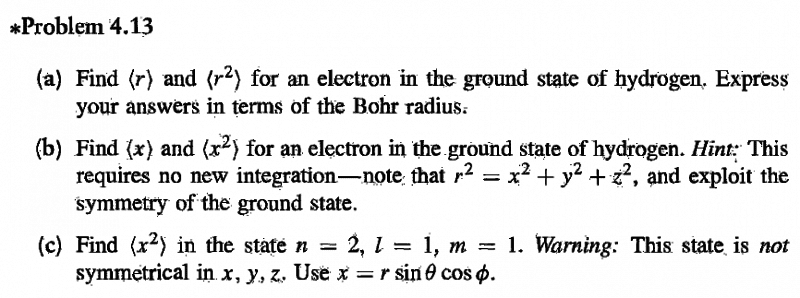# Expectation values r and x for electron in H2 ground state

Gold Member

## Homework Statement## Homework Equations

$$\psi_{100} = \frac {1}{\sqrt{\pi a^{3}}} e^{-r/a}$$

## The Attempt at a Solution

a)
$$\langle r \rangle = \frac {1}{\pi a^{3}} \int_0^{2 \pi} d \phi \int_{0}^\pi d \theta \int_0^{\infty} r^{3} e^{-2r/a} dr$$
This comes out to be ##\frac {3}{2}a##

$$\langle r^{2} \rangle = \frac {1}{\pi a^{3}} \int_0^{2 \pi} d \phi \int_{0}^\pi d \theta \int_0^{\infty} r^{4} e^{-2r/a} dr$$
Which comes out as ##3a^{2}##

b)
I know ##r^{2} = 3x^{2}##, so the answer for the expectation value of ##x^{2}## is one third the expectation value of ##r^{2}##, therefore ##\langle x^{2} \rangle = a^{2}##

However, I am confused how to find ##\langle x \rangle##. Do I just say ##x = r sin \theta##, therefore ##dx = sin \theta dr + r cos \theta d \theta##?

Last edited:

mfb
Mentor
<x> is easy to find by symmetry, but you can calculate the integral if you want.

Gold Member
Yes, I am confused how to exploit the symmetry

mfb
Mentor
Do you expect the electron to be on average more on the left or the right side? Does that question even make sense as you don't know where left and right are?

Gold Member
Okay, I suppose that makes sense, but then why would the square x coordinate not be zero then? By the same reasoning

PeroK